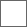• 115回复贴，共1

### 我的自然存在哲学的思维进化W=ρV
W=∰ρdVEz=ζWz

σo=Ez/Vz=ζWz/Vz=ζρoEd=α×Eo=(u/c)×Eo=(ω×r/c)×Eo

F=q(Eδ+β×Ed)/γH=dP/dt=d(WU)/dt

H=dP/dt=d(WU)/dt=UdW/dt=UQ=ρSUU

H=-H˙

F=-F˙@平阳睡狮郭峰君 一个正常辩论能被删的贴吧能被称为“科学的自由天空”吗？

@绝对运动6 你说过宇宙空间要是能扭曲的话就会降成一维二维是吗？Ed=WUU

Ek1=WVV

Ed-Ek1=W(UU-VV)

Ek2=0#### 扫二维码下载贴吧客户端